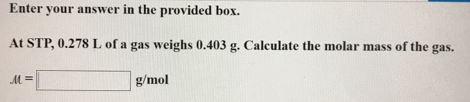# Problem: At STP, 0.278 L of a gas weighs 0.403 g. Calculate the molar mass of the gas. M = _______ g/mol

###### FREE Expert Solution
81% (280 ratings)###### Problem Details

At STP, 0.278 L of a gas weighs 0.403 g. Calculate the molar mass of the gas.

M = _______ g/mol